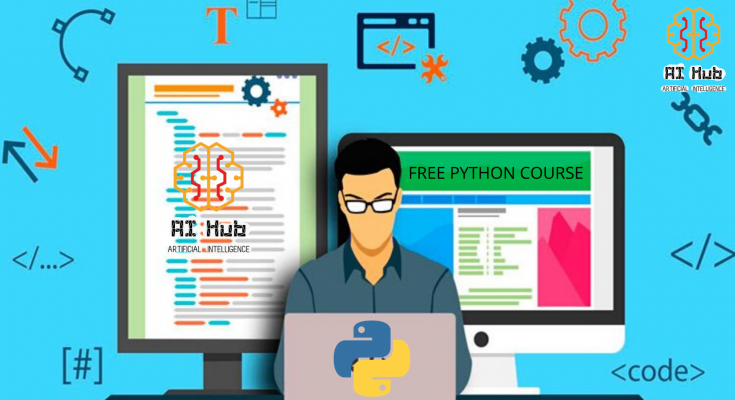# FUNCTION PROGRAMS IN PYTHON##### EXAMPLE 01: ADD TWO NUMBERS
``````>>> def add(x,y):
return(x+y)

>>> a = 3
>>> b = 5
>>> print ( "sum of ",a," & ",b," is ",c)

Output:
sum of 3 & 5 is 8``````
``````>>> def add(x,y):
return(x+y)
>>> a = int(input ("enter first number "))
>>> b = int(input ("enter second number "))
>>> print ( "sum of ",a," & ",b," is ",c)

Output:
enter first number 5
enter second number 6
sum of  5  &  6  is  11``````
##### EXAMPLE 02: AREA OF RECTANGLE
``````>>> def area(l,b):
return(l*b)

>>> l = int(input ("enter length "))
>>> b = int(input ("enter breadth "))
>>> a = area(l,b)
>>> print("area of rectangle is ",a)

Output:
enter length 5
area of rectangle is  30``````
##### EXAMPLE 03: ODD OR EVEN NUMBER
``````>>> def oe(num):
if (num%2==0):
print(num," is even number")
else:
print(num," is odd number")

>>> num = int(input ("enter a number : "))
>>> oe(num)

Output:
enter a number : 4
4  is even number``````
##### EXAMPLE 04: CHECK PRIME NUMBER
``````>>> def prime(a):
c = 0
if (a<1):
print("invalid input ! Try next number ")
elif(a==1):
print ("1 is not prime")
else:
for i in range(2,a,1):
if (a%i==0):
c=1
if (c==0):
print(a," is prime")
else:
print(a," is not prime")

>>> num = int (input("enter a number : "))
>>> prime(num)

Output:
enter a number: 6
6  is not prime``````
##### EXAMPLE 05: AREA OF RECTANGLE USING LAMBDA
``````#>>> area = (lambda x,y : x*y)(5 , 4)     next method
>>> area = (lambda x,y : x*y)
>>> print ( area(5,4))

Output:
Area of rectangle is : 20``````

This page is contributed by Diwas & Sunil . If you like AIHUB and would like to contribute, you can also write an article & mail your article to  itsaihub@gmail.com . See your articles appearing on AI HUB platform and help other AI Enthusiast.🚀 I'm Diwas Pandey, a Computer Engineer with an unyielding passion for Artificial Intelligence, currently pursuing a Master's in Computer Science at Washington State University, USA. As a dedicated blogger at AIHUBPROJECTS.COM, I share insights into the cutting-edge developments in AI, and as a Freelancer, I leverage my technical expertise to craft innovative solutions. Join me in bridging the gap between technology and healthcare as we shape a brighter future together! 🌍🤖🔬

View all posts by Diwas →

### 4 Comments on “FUNCTION PROGRAMS IN PYTHON”

1.website host says:

If you wish for to increase your knowledge only keep visiting this web page and be updated with the latest news posted here.

2.web hosting sites says:

We’re a group of volunteers and opening a new scheme in our community.
Your website offered us with valuable info to work on. You’ve done an impressive job
and our entire community will be thankful to
you.